#Quality Digest

Featured Product
This Week in Quality Digest Live
Statistics Features
Donald J. Wheeler
What do predictable processes have in common with chaos?
William A. Levinson
People can draw the wrong conclusions due to survivor, survey, and bad news bias.
Donald J. Wheeler
Does your approach do what you need?
Paul Laughlin
Correlation vs. causality
Donald J. Wheeler
In spite of what everyone says to the contrary
Statistics News
New capability delivers deeper productivity insights to help manufacturers meet labor challenges
Day and a half workshop to learn, retain, and transfer GD&T knowledge across an organization
Elsmar Cove is a leading forum for quality and standards compliance
InfinityQS’ quality solutions have helped cold food and beverage manufacturers around the world optimize quality and safety
User friendly graphical user interface makes the R-based statistical engine easily accessible to anyone
Collect measurements, visual defect information, simple Go/No-Go situations from any online device
Good quality is adding an average of 11 percent to organizations’ revenue growth
Ability to subscribe with single-user minimum, floating license, and no long-term commitment
A guide for practitioners and managersBio

Statistics

## More About the Precision to Tolerance Ratio

### What does it tell us about the usefulness of a measurement?

Published: Tuesday, June 1, 2021 - 12:03

For more than 40 years it has been common to use the precision to tolerance ratio (P/T ratio) to compare the standard deviation of measurement error with the specified tolerance for a particular product. The purpose of this comparison being an assessment of the utility of the measurement process. Here we shall look at what the P/T ratio does and does not do.

### The structure of a product measurement

We will need some notation for what follows. So, let the product measurements be denoted by X. These product measurements may be thought of as consisting of two components. The value of the item being measured may be denoted by Y, while the error of the measurement may be denoted by E. Thus: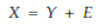If we think about these quantities as variables, then the variation in the stream of product measurements, Var(X), will consist of the variation in the stream of product values, Var(Y), plus the variation in the stream of measurement errors, Var(E).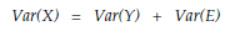In practice it is the product measurements, X, that are compared with the specifications. Since measurement error, E, is only one component of a product measurement it follows that any comparison between measurement error and the specified tolerance can only tell part of the story.

But what is the story that needs to be told? It generally takes the form of “Is the measurement system good enough to let us make and ship good product?” And the answer to this question involves more than just the measurement error and the specified tolerance.

To determine if we can make and ship good product, we will also need to characterize the production process. But any attempt to characterize a production process implicitly assumes that the process is being operated predictably. For unless a process is operated predictably the notions of process location and process dispersion are not well-defined. These notions have no fixed values when the process is changing without warning.

So, while we may always compute descriptive statistics from our data, and while these statistics will always describe the past, these statistics cannot be used to estimate process parameters until the notion of a process parameter makes sense, and this only happens when a process is operated predictably.

Consequently, when we attempt to characterize a production process we are talking about what can be achieved when the process is operated predictably and on-target. For it is only in this latter case that the actual process potential approaches the hypothetical.

When a process is operated off-target it will not achieve its full potential due to an increased fraction nonconforming or an increased amount of “give away.” Likewise, when a process is operated unpredictably it will fail to achieve its full potential because of increased variation in the process stream.

Nevertheless, because it is useful to understand the hypothetical process potential, we shall assume our process is operated predictably and on-target in what follows.

### Hypothetical process yield

When the capability ratio is less than 1.00 there will generally be some nonconforming product produced. Here the product produced will fall into one of four categories: good product that is shipped, good product that is rejected, bad product that is shipped, and bad product that is rejected. In the interest of simplicity I shall use the number of good parts shipped per thousand parts produced as a measure of the hypothetical yield of the process.

There are three quantities that determine the hypothetical yield of a predictable process that is centered within the specifications. These are the variation in the stream of product measurements; the variation in the measurement process; and the specified tolerance. The variation in the stream of product measurements, X, may be characterized by either SD(X) or Var(X). The specified tolerance will be defined as the difference between the upper watershed specification limit and the lower watershed specification limit. And the variation in the measurement process may be characterized by either SD(E) or Var(E). All three of these components act together to determine the hypothetical process yield.Figure 1: Three quantities determine the hypothetical process potential

Now we already know how to compare these three quantities. The capability ratio, Cp, compares the specified tolerance with the standard deviation of the product measurements: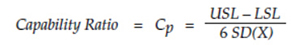The precision to tolerance ratio compares the standard deviation of the measurement process with the specified tolerance.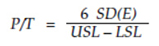The intraclass correlation coefficient (ICC) defines that proportion of the variation in the stream of product measurements, Var(X), that can be directly attributed to the variation in the product steam, Var(Y)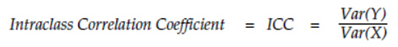This makes [ 1 –  ICC ] the correct and appropriate comparison between the variance of the measurement process, Var(E), and the variance of the stream of product measurements, Var(X)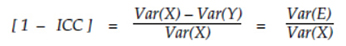Figure 2: Comparisons commonly used to characterize yields

These three comparisons are all related in the manner shown by the following equation. This means that knowledge of any two of these components will completely define the third.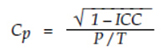So, while any two of these three components will tell the whole story, the nonlinear relationships between them complicate the way these components are related to the process yields. For example, we usually think of capability as determining the yield as shown in figure 3.Figure 3: Traditional curve for capability vs. yield

This representation of the relation between capability and yield ignores the impact of measurement error. It assumes that the ICC value is 1.00 (and that measurement error is therefore zero). When we take measurement error into account we get figure 4. The four curves show the effect of capability at different ICC values. From top to bottom these curves have intraclass correlations of 0.995, 0.80, 0.60, and 0.40. The vertical lines show the effect of measurement error at a given capability.Figure 4: Hypothetical yield depends on both capability and ICC

Here we see that the intraclass correlation has a negligible or minimal impact upon yield when the capability ratio exceeds 1.20. However, measurement error has an increasingly detrimental effect upon yield as the capability drops below 1.00.

Since the capability ratio and intraclass correlation completely determine a P/T ratio, we can show the relationship between all four variables by mapping the curves of figure 4 as a function of P/T and yield. This results in figure 5.Figure 5: The relationship between yield, P/T, capability, and ICC

The “horizontal” red lines connect points having the same capability ratio. Capabilities of 0.2, 0.3, 0.4, 0.5, 0.6, and 0.7 are shown.

The “vertical” blue curves connect points having the same intraclass correlation. ICC values of 0.995, 0.98, 0.96, 0.9, 0.8, 0.7, 0.6, and 0.5 are shown.

The envelope defined by the curve for ICC = 0.995 and the curve for ICC = 0.50 contains all of the possible combinations of yield and P/T ratios. (The curves for smaller intraclass correlations all fall within the envelope shown and so were omitted for clarity.) Combinations of yield and P/T to the left of the 0.995 curve are impossible. Likewise, combinations above the ICC = 0.50 curve are also impossible.

### What can we learn from figure 5?

While a P/T ratio cannot, by itself, determine the process yield, when the P/T ratio is less than 0.10 we can place a lower bound of 950 ppt on the hypothetical yield. So having a small P/T ratio is a good sign that your process has the potential for a high yield.

However, having larger P/T ratios does not immediately limit your process potential. Potential yields can be as high as 1000 ppt for P/T ratios as large as 0.45, and they can be in excess of 950 ppt for P/T ratios as large as 0.75. Thus, figure 5 completely undermines the widespread idea that P/T ratios in excess of 0.3 can be used to condemn a measurement process. A small P/T ratio may be good, but that does not mean that a large P/T ratio is bad.

### Summary

The hypothetical process yield can be determined from any two of the three ratios listed in figure 2. The relationship between the process yield and the capability ratio is well-known and understood (for the case where measurement error is zero). This relationship was shown in figure 3.

When we include the effects of measurement error we end up with figure 4. Here, for a given capability, the yield will drop as the ICC drops. However, the impact of measurement error becomes less important as the capability increases. When the capability ratio exceeds 1.2 the impact of measurement error becomes negligible. Thus, the relationship shown in figure 4 between yield, capability, and the intraclass correlation is easy to understand.

However, if we seek to pair the P/T ratio with either the capability ratio or the intraclass correlation in order to characterize the process yield, then we will have to use the convoluted relationships shown by the curves in figure 5. When we do this we find that there is no simple way to describe how the yields vary with the P/T ratio. The P/T ratio is simply too blunt an instrument to provide any meaningful assessment of the utility of a measurement process.

So we still have three ratios that can be used in pairs to determine the potential process yield: these ratios are the capability ratio; the intraclass correlation; and the precision to tolerance ratio. And by far the least useful of these three is the precision to tolerance ratio.

### Discuss